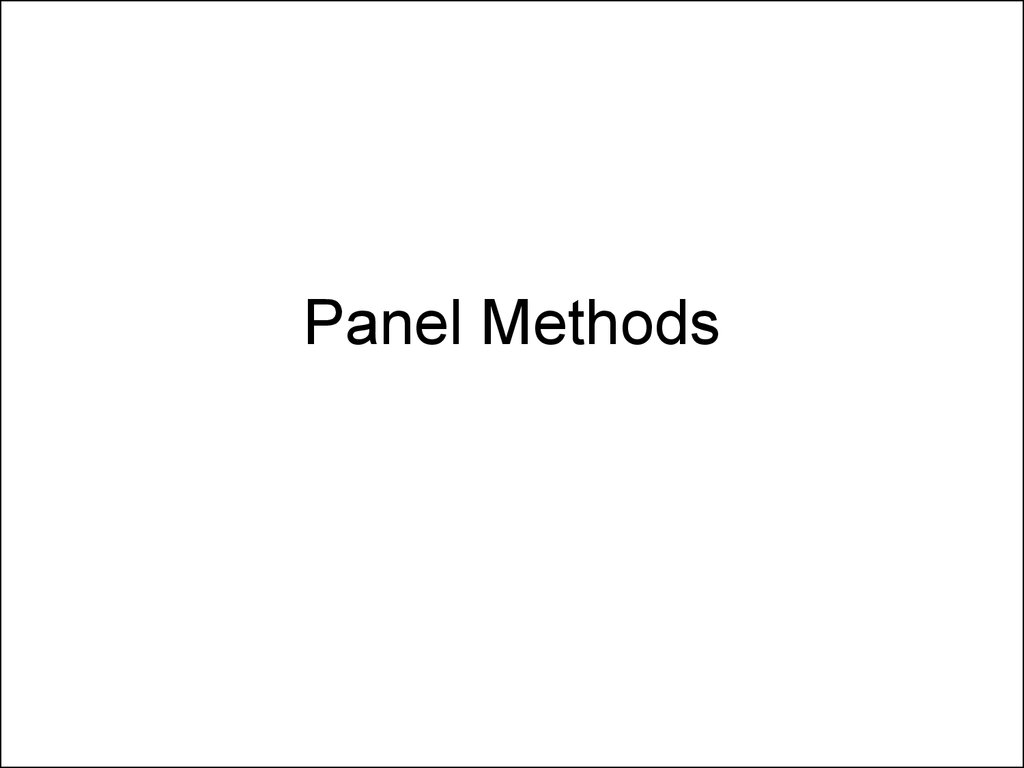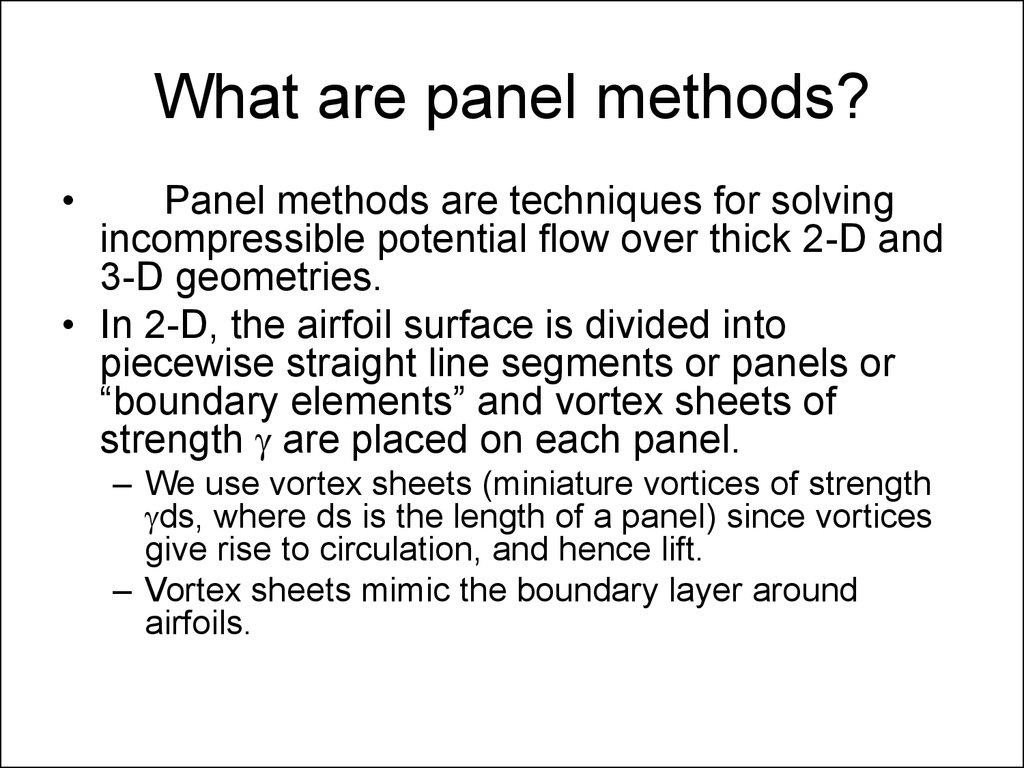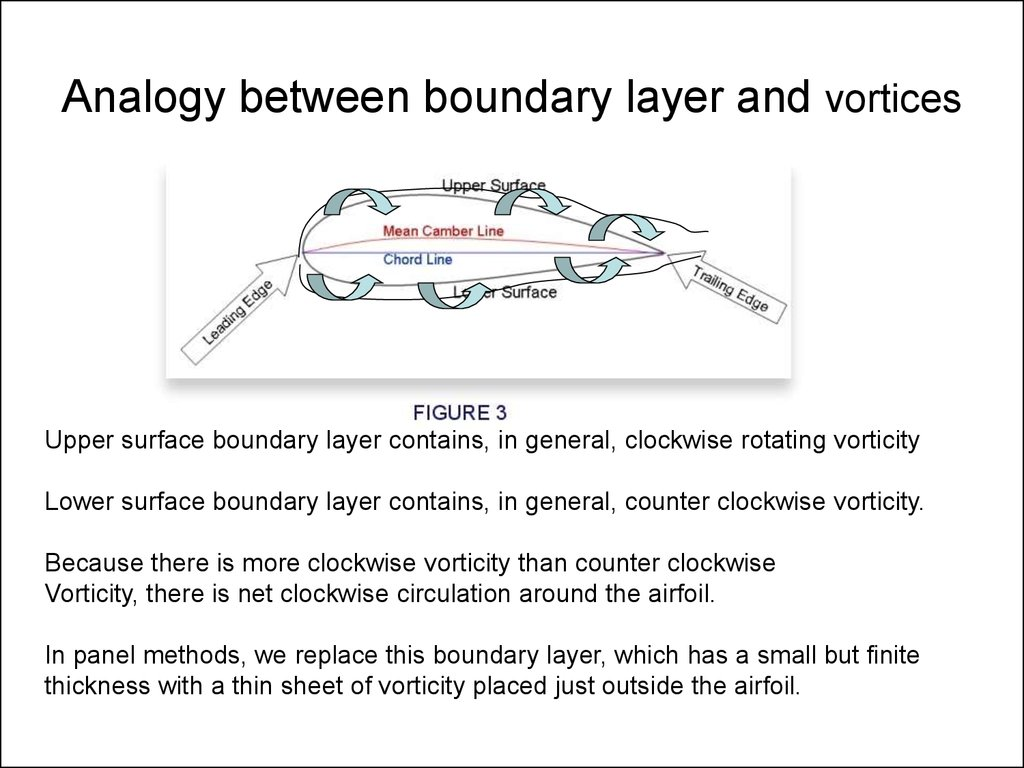Panel.Methods

2. What are panel methods?

Panel methods are techniques for solving
incompressible potential flow over thick 2-D and
3-D geometries.
• In 2-D, the airfoil surface is divided into
piecewise straight line segments or panels or
“boundary elements” and vortex sheets of
strength g are placed on each panel.
– We use vortex sheets (miniature vortices of strength
gds, where ds is the length of a panel) since vortices
give rise to circulation, and hence lift.
– Vortex sheets mimic the boundary layer around
airfoils.

3. Analogy between boundary layer and vortices

Upper surface boundary layer contains, in general, clockwise rotating vorticity
Lower surface boundary layer contains, in general, counter clockwise vorticity.
Because there is more clockwise vorticity than counter clockwise
Vorticity, there is net clockwise circulation around the airfoil.
In panel methods, we replace this boundary layer, which has a small but finite
thickness with a thin sheet of vorticity placed just outside the airfoil.

4. Panel method treats the airfoil as a series of line segments

On each panel, there is vortex sheet of strength DG = g0 ds0
Where ds0 is the panel length.
Each panel is defined by its two end points (panel joints)
and by the control point, located at the panel center, where we will
Apply the boundary condition y= Constant=C.
The more the number of panels, the more accurate the solution,
since we are representing a continuous curve by a series
of broken straight lines

5. Boundary Condition

• We treat the airfoil surface as a streamline.
– This ensures that the velocity is tangential to the
airfoil surface, and no fluid can penetrate the surface.
• We require that at all control points (middle
points of each panel) y= C
• The stream function is due to superposition of
the effects of the free stream and the effects of
the vortices g0 ds0 on each of the panel.

6. Stream Function due to freestream

• The free stream is given by
Recall
y
u;
y
y
v
y
This solution satisfies conservation of mass
And irrotationality
u y v x
u
v
0
x
y
u
v
0
y
x
It also satisfies the Laplace’s equation. Check!

y
1 y
0
r
y
G
v
r
2 r
vr
vr 0
v
G
2 r
Circulation
G
ln r
2
v rd G

8. Stream function Vortex, continued..

• Pay attention
to the signs.
• A counterclockwise
vortex is
considered
“positive”
• In our case,
the vortex of
strength g0ds0
placed on a
panel with
location (x0
and y0).
• Then the
stream
function at a
point (x,y) will
be
g 0 ds0
y
ln r ro
2
where
r r x x y y
2
o
0
2
0
Control Point whose center
point is (x,y)
Panel whose center
point is (x0,y0)

9. Superposition of All Vortices on all Panels

• In the panel method we use here, ds0 is the length of a
small segment of the airfoil, and g0 is the vortex strength
per unit length.
• Then, the stream function due to all such infinitesimal
vortices at the control point (located in the middle of
each panel) may be written as the interval below, where
the integral is done over all the vortex elements on the
airfoil surface.
g0
ln r r0 ds0
2

10. Adding the freestream and vortex effects..

1
u y v x
g
ln
r
r
ds
C
0
0
o
2
The unknowns are the vortex strength g0 on each panel, and the value of the
Stream function C.
Before we go to the trouble of solving for g0, we ask what is the purpose..

11. Physical meaning of g0

V = Velocity of the flow just outside the boundary layer
Panel of length ds0 on the airfoil
Sides of our contour
have zero height
Bottom side has zero
Tangential velocity
Because of viscosity
Its circulation = DG g0 ds0
V ds g 0 ds0 Vds0
Circulatio n
Contour
Or ,
V g 0
If we know g0 on each panel, then we know the velocity of the flow
outside the boundary layer for that panel, and hence pressure over that panel.

1
1
2
2
Bernoulli says : p u v p V 2
2
2
p p
u2 v 2
V2
Cp
1
1 2
2
1
V
V
2
V
2
Since V = -g0
Cp 1
g
V
2
0
2

13. Kutta Condition

• Kutta condition states that the pressure above and below the airfoil
trailing edge must be equal, and that the flow must smoothly leave
the trailing edge in the same direction at the upper and lower edge.
g2upper = V2upper
g2lower = V2lower
F
From this sketch above, we see that pressure will be equal, and the flow
will leave the trailing edge smoothly, only if the voritcity on each panel
is equal in magnitude above and below, but spinning in opposite
Directions relative to each other.
g Upper g lower

14. Summing up..

• We need to solve the integral equation derived
earlier
• And, satisfy Kutta condition.
1
u y v x
g 0 ln r r0 dso C
2
g Upper g lower

15. Numerical Procedure

• We divide the airfoil into N panels. A typical panel is given the
number j, where J varies from 1 to N.
• On each panel, we assume that g0 is a piecewise constant. Thus, on
a panel numbered j, the unknown strength is g0,j
• We number the control points at the centers of each panel as well.
Each control point is given the symbol “i”, where i varies from 1 to N.
• The integral equation becomes
g 0, j
u yi v xi
ln ri r0 dso C 0
j 1.. N 2 j

16. Numerical procedure, continued

• Notice that we use two indices ‘i’ and ‘j’. The index ‘I’
refers to the control point where equation is applied.
• The index ‘j’ refers to the panel over which the line
integral is evaluated.
• The integrals over the individual panels depends only on
the panel shape (straight line segment), its end points
and the control point í’.
• Therefore this integral may be computed analytically.
• We refer to the resulting quantity as
N
u yi v xi Ai , jg 0 j C 0
where,
j 1
1
Ai , j Influence of Panel j on index i =
ln ri r0 ds0
2

17. Numerical procedure, continued..

• We thus have N+1 equations for the unknowns g0,j (j=1…N)
and C.
• We assume that the first panel (j=1) and last panel (j=N) are
on the lower and upper surface trailing edges.
N
u yi v xi Ai , j g 0 j C 0
j 1
g 0,1 g 0, N
This linear set of equations may be solved easily, and g0 found.
Once go is known, we can find pressure, and pressure coefficient Cp.

18. Panel code

• Our web site contains a Matlab code I have written, if
you wish to see how to program this approach in Matlab.
• See
http://www.ae.gatech.edu/people/lsankar/AE3903/Panel.
m
• And, sample input file
http://www.ae.gatech.edu/people/lsankar/AE3903/panel.
data.txt
• An annotated file telling you what the avrious numbers in
the input means is found at
– http://www.ae.gatech.edu/people/lsankar/AE3903/Panel.Code.In
put.txt

19. PABLO

• A more powerful panel code is found on the
web.
• It is called PABLO: Potential flow around Airfoils
with Boundary Layer coupled One-way
• See